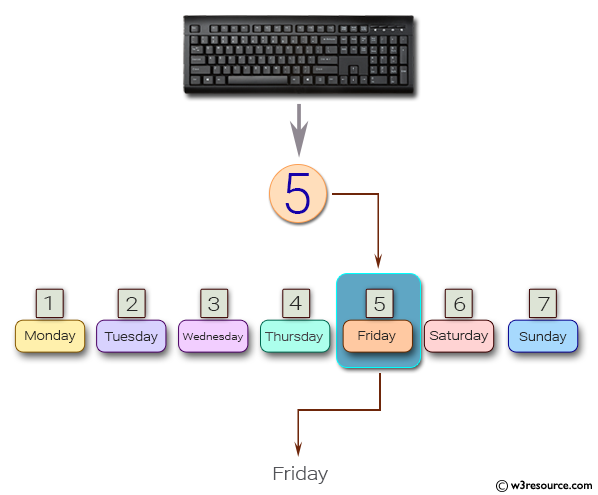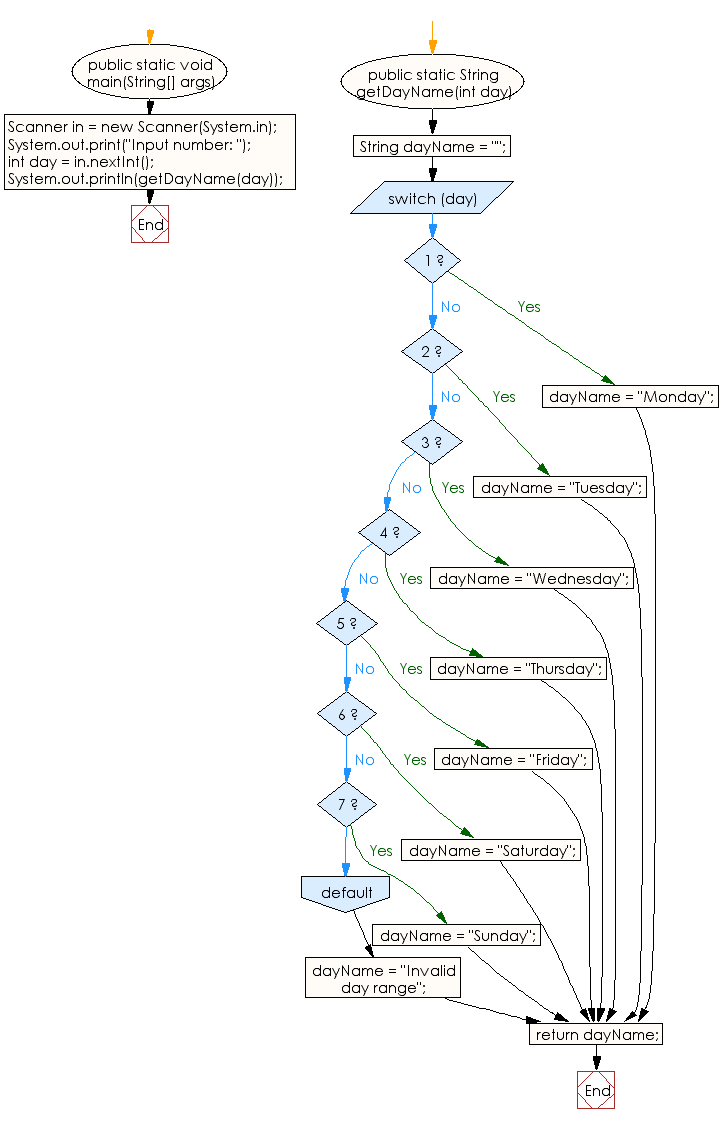﻿ Java - Displays the weekday between 1 and 7

# Java: Displays the weekday between 1 and 7

## Java Conditional Statement: Exercise-5 with Solution

Write a Java program that takes a number from the user and generates an integer between 1 and 7. It displays the weekday name.

Test Data
Input number: 3

Pictorial Presentation:Sample Solution:

Java Code:

``````import java.util.Scanner;
public class Exercise5 {

public static void main(String[] args)
{
Scanner in = new Scanner(System.in);
System.out.print("Input number: ");
int day = in.nextInt();

System.out.println(getDayName(day));
}

// Get the name for the Week
public static String getDayName(int day) {
String dayName = "";
switch (day) {
case 1: dayName = "Monday"; break;
case 2: dayName = "Tuesday"; break;
case 3: dayName = "Wednesday"; break;
case 4: dayName = "Thursday"; break;
case 5: dayName = "Friday"; break;
case 6: dayName = "Saturday"; break;
case 7: dayName = "Sunday"; break;
default:dayName = "Invalid day range";
}

return dayName;
}
}
```
```

Sample Output:

```Input number: 3
Wednesday
```

Flowchart:Java Code Editor:

What is the difficulty level of this exercise?

Test your Programming skills with w3resource's quiz.

﻿

## Java: Tips of the Day

IsPowerOfTwo

Checks if a value is positive power of two.

To understand how it works let's assume we made a call IsPowerOfTwo(4).

As value is greater than 0, so right side of the && operator will be evaluated.

The result of (~value + 1) is equal to value itself. ~100 + 001 => 011 + 001 => 100. This is equal to value.

The result of (value & value) is value. 100 & 100 => 100.

This will value the expression to true as value is equal to value.

```public static boolean isPowerOfTwo(final int value) {
return value > 0 && ((value & (~value + 1)) == value);
}
```

Ref: https://bit.ly/3sA5d4I

We are closing our Disqus commenting system for some maintenanace issues. You may write to us at reach[at]yahoo[dot]com or visit us at Facebook